## Getting Started:¶

This is an IPython notebook. IPython notebooks are open-source programming tools that run in a web browser, and offer a lot of flexibility and power for scientific computing tasks. Because they are portable and self-contained, they are ideal for education and reproducable research. Notebooks are made of a single column of 'cells' that usually consist of markdown or code. Markdown cells like this one and the one above support basic markdown formatting, as well as $\LaTeX$ statements. The cell below is a code cell, which runs Python code interactively if you use your personal computer or a cloud service like Domino. The Python commands can be run over and over, or the background processes can be stopped or restarted without affecting this notebook. Did I mention flexibility? Cells can be reordered, and the whole notebook can be saved as a PDF document, or can be hosted as a static webpage on sites like nbviewer.

Scientific applications of the Python progamming language have increased dramatically over the past few years. Its simplicity, versatility, and power has made it a first-choice language for many scientists and engineers. Just enter "import antigravity" in a code cell or at a Python prompt to see the zeal Python inspires.

To get started, the main function libraries in Python for working with numbers are numpy and pandas. Standard numerical methods are often in the scipy library. The sklearn library has fitting and regression, and matplotlib is used for matlab-style plots. To begin our Python session, these libraries and some specific functions are imported in the cell below:

In :
import numpy as np
import pandas as pd
from scipy.optimize import fsolve
from scipy.optimize import curve_fit
from scipy.integrate import odeint

from sklearn.pipeline import make_pipeline
from sklearn.preprocessing import PolynomialFeatures
from sklearn.linear_model import LinearRegression

import matplotlib.pyplot as plt
# turn on inline plotting for this notebook:
%matplotlib inline


# Reference¶

This hastily written notebook attempts to replicate the workflow and results of M. Shacham's "Using Templates for Demonstrating Good Programming Practices" submitted to the 2015 Annual AIChE meeting: ftp site

# Basic Numerical Problem Solving with Python¶

## One Nonlinear (Implicit) Algebraic Equation¶

Solve the followng system of equations:

$$P=\frac{RT}{\left(V-b\right)}-\frac{\alpha a}{V\left(V+b\right)}$$

where:

$$a=0.42747\left(\frac{R^2T_c^2}{P_c}\right)$$$$b=0.08664\left(\frac{RT_c}{P_c}\right)$$$$m=0.48508+1.55171\bar{\omega}-0.1561\bar{\omega}^2$$$$\alpha=\left[1+m\left(1-\sqrt{T/T_c}\right)\right]$$

Create a Python function for the first equation, set equal to zero:

In :
def f(V):
return R*T/(V-b)-alph*a/(V*(V+b))-P


Enter some constants

In :
R=0.08206 #Gas constant (L-atm/gmol-K)
P_c=72.9
T_c=304.2
w_bar=0.225
T=300.
P=100.
a=0.42747*(R**2.0*T_c**2.0/P_c)
b=0.08664*(R*T_c/P_c)
m=0.48508+1.55171*w_bar-0.1561*w_bar**2
alph=(1+m*(1-np.sqrt(T/T_c)))**2


Print out values, and solve equation with initial guess

In :
print('initial f(V0)= %f' %f(0.07))
print('a = %f' %a)
print('b = %f' %b)
print('m = %f' %m)
print('alpha = %f' %(alph))
print('V= %f' %(fsolve(f,0.07))) # Print out solution result

initial f(V0)= -19.366385
a = 3.653924
b = 0.029668
m = 0.826312
alpha = 1.011481
V= 0.064113


The final result of the optimization is $V = 0.0641$

## A System of Nonlinear Algebraic Equations (NLE)¶

The following is a system of ultrafiltration equations from Cutlip and Shackam, 2013

$$Q_1c_1=Q_0c_0$$$$Q_1=Q_0-j_1A$$$$j_1=k\ln\left(c_g/c_1\right)$$$$Q_2c_2=Q_1c_1$$$$j_2=k\ln\left(c_g/c_2\right)$$$$Q_3c_3=Q_2c_2$$$$Q_3=Q_2-j_3A$$$$j_3=k\ln\left(c_g/c_3\right)$$$$Q_0=1/(60\times1000)$$$$k=3.5e-6$$
In :
cg=300
c0=10
A=0.9
k=3.5e-6
Q0=1/(60.0*1000.0)
Const=[cg,c0,A,k,Q0]
xguess=[40.0,70.0,150.0]

def Evarfun(x,Const):
c1,c2,c3 = x # unpack concentrations
cg,c0,A,k,Q0 = Const # unpack constants
j1=k*np.log(cg/c1)
Q1=c0*Q0/c1
j2=k*np.log(cg/c2)
Q2=c1*Q1/c2
j3=k*np.log(cg/c3)
Q3=c2*Q2/c3
Evar=[j1,j2,j3,Q1,Q2,Q3]
return Evar

def MNLEfun(x,Const):
c1,c2,c3=x# unpack concentrations
cg,c0,A,k,Q0 = Const # unpack constants
j1=k*np.log(cg/c1)
Q1=c0*Q0/c1
j2=k*np.log(cg/c2)
Q2=c1*Q1/c2
j3=k*np.log(cg/c3)
Q3=c2*Q2/c3
fx=[Q0-Q1-(j1*A),Q1-Q2-(j2*A),Q2-Q3-(j3*A)]
return fx


Check function values at initial guesses:

In :
evars_initial=Evarfun(xguess,Const)
var_names=['j1: ','j2: ','j3: ','Q1: ','Q2: ','Q3: ']
for var in zip(var_names,evars_initial):
print(var+'{:.2e}'.format(var))

j1: 7.05e-06
j2: 5.09e-06
j3: 2.43e-06
Q1: 4.17e-06
Q2: 2.38e-06
Q3: 1.11e-06


OK, it is time to solve, note that the solver tolerance xtol must be reduced due to the very small function values:

In :
c=fsolve(MNLEfun,xguess,args=Const,xtol=1e-13)
print('c1 = %.2f \nc2 = %.2f \nc3 = %.2f' %(c,c,c))
print('function values = ' + str(MNLEfun(c,Const)))
evars_final=Evarfun(c,Const)
for var in zip(var_names,evars_final):
print(var+'{:.2e}'.format(var))

c1 = 20.35
c2 = 56.67
c3 = 163.12
function values = [0.0, 0.0, -4.2351647362715017e-22]
j1: 9.42e-06
j2: 5.83e-06
j3: 2.13e-06
Q1: 8.19e-06
Q2: 2.94e-06
Q3: 1.02e-06


We are able to solve the equations for the values of $c_1,c_2$ and $c_3$ , note that the objective function values are all very nearly zero, indicating that we have accurately fit our model. The $c$ values as well as the $Q$ and $j$ values now fully describe the unit operation.

## Systems of First-Order Ordinary Differential Equations (ODEs) - Initial Value Problems (IVPs)¶

Multicomponent gas diffusion is described by the following system of equations:

$$\frac{dC_A}{dz}=\frac{x_AN_B-x_BN_A}{D_{AB}}+\frac{x_AN_C-x_CN_A}{D_{AC}}$$$$\frac{dC_B}{dz}=\frac{x_BN_A-x_AN_B}{D_{AB}}+\frac{x_BN_C-x_CN_B}{D_{BC}}$$$$\frac{dC_C}{dz}=\frac{x_CN_A-x_AN_C}{D_{AC}}+\frac{x_CN_B-x_BN_C}{D_{BC}}$$$$x_A=C_A/C_T$$$$x_B=C_B/C_T$$$$x_C=C_C/C_T$$$$N_A=2.115\times10^{-4}$$$$N_B=-4.143\times10^{-4}$$$$N_C=0$$$$D_{AB}=1.47\times10^{-4}$$$$D_{AC}=1.075\times10^{-4}$$$$D_{BC}=1.245\times10^{-4}$$$$C_T=0.2/(0.082057\times328)$$

Define the function that calculates the derivatives at any point in time

In :
def ODEfun(Yfunvec,z,NA,NB,NC,DAB,DBC,DAC,CT):
CA,CB,CC=Yfunvec
xA=CA/CT
xB=CB/CT
xC=CC/CT
dCBdz=(xB*NA-(xA*NB))/DAB+(xB*NC-(xC*NB))/DBC
dCCdz=(xC*NA-(xA*NC))/DAC+(xC*NB-(xB*NC))/DBC



Now, define the constants and run the integration

In :
NA=0.00002115
NB=-0.0004143
NC=0
DAB=0.000147
DBC=0.0001245
DAC=0.0001075
CT=0.2/(0.082057*328)
Const=NA,NB,NC,DAB,DBC,DAC,CT

y0=[0.00022290,0,0.007208]
tspan=np.linspace(0,0.001,40)
ty=odeint(ODEfun,y0,tspan,args=Const)


Plot the results:

In :
plt.plot(tspan,ty)
plt.legend(['$C_A$','$C_B$','$C_C$'])
plt.xlabel('z (m)')
plt.ylabel('Dependent variable')

Out:
<matplotlib.text.Text at 0x7fe928e66ed0>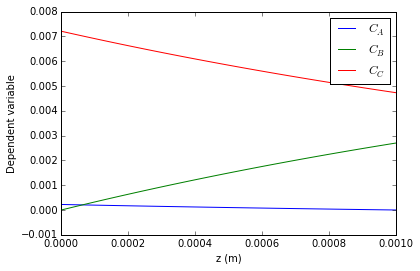Using our ODEs, we have described the gas concentrations over distance for the diffusion problem.

## Linear and Polynomial Regression¶

Regressing model parameters for a linear or polynomial equation is very common and straightforward. The goal in each case is determining the parameter values $b_0, b_1...b_n$ given a set of observations $y_i$ at different inputs $x_i$.

The form of a linear equation is:

$$\hat{y_i}=b_0+b_1x_i$$

For a polynomial:

$$\hat{y_i}=b_0+b_1x_i+b_2x_i^2+...+b_nx_i^n$$

Where $\hat{y_i}$ is the calculated observation. The goal of the regression is to find values of $b$ so that $\hat{y_i}$ matches $y_i$ for each of the values of $x_i$. To do regression, we need data, which is typically a table. For working with tables of data, the python pandas library is indespensible

In :
# input data points
T=[290.08, 302.39, 311.19, 318.69, 325.1, 330.54, 334.89, 338.94,
342.95, 346.24, 349.91, 353.47, 356.19, 358.87, 362.29, 365.23,
367.9, 370.53, 373.15, 375.84, 378.52, 381.32,]
P=[8634, 15388, 22484, 30464, 38953, 47571, 55511, 63815, 72985,
81275, 91346.0, 102040, 110850, 120140, 132780, 144530, 155800,
167600, 180060, 193530, 207940, 223440]

# convert to data table- first stick T to P, then transpose and add labels
data=pd.DataFrame(np.transpose(np.vstack((T,P))),columns=['Temperature','Pressure'])
data # print out data table

Out:
Temperature Pressure
0 290.08 8634
1 302.39 15388
2 311.19 22484
3 318.69 30464
4 325.10 38953
5 330.54 47571
6 334.89 55511
7 338.94 63815
8 342.95 72985
9 346.24 81275
10 349.91 91346
11 353.47 102040
12 356.19 110850
13 358.87 120140
14 362.29 132780
15 365.23 144530
16 367.90 155800
17 370.53 167600
18 373.15 180060
19 375.84 193530
20 378.52 207940
21 381.32 223440
In :
# Temperature and pressure are changed to column vectors for sklearn
X=data['Temperature'].values[:,np.newaxis]
y=data['Pressure'].values[:,np.newaxis]
model=make_pipeline(PolynomialFeatures(degree=3), LinearRegression())
model.fit(X,y)
yhat=model.predict(X)


Make plot of predicted vs. measured pressures, get your magnifying glasses...

In :
plt.plot(X,y,'b*',label='Experimental')
plt.plot(X,y,'g+',label='Calculated')
plt.legend(loc='best')
plt.xlabel('Temp (K)')
plt.ylabel('Press. (Pa)')
plt.show()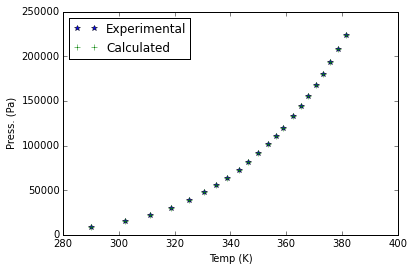The experimental and calculated data points nearly overlap!

The plot of the residuals shows more diagnostics- the fact that these residual points are not randomly distributed indicates the equation we are fitting is not ideal:

In :
plt.plot(y,y-yhat,'b*')
plt.xlabel('Press. (measured, Pa)')
plt.ylabel('Residual')
plt.title('RMSE=%.1f Pa' %(np.sqrt(sum((y-yhat)**2))))

Out:
<matplotlib.text.Text at 0x7fe9260b1250>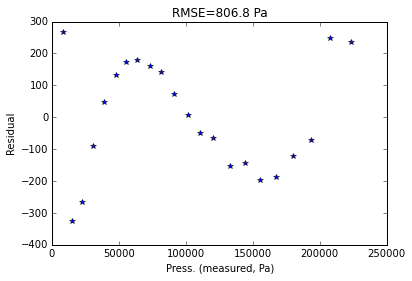The results from the polynomial fitting show a non-random trend in the residuals- this model is not ideal to describe the data...

To use the Riedel equation, which is a linear combination of some (nonlinear) treaments of the Temperature data:

$$\log(P)=b_0+\frac{b_1}{T}+b_2\log(T)+b_3T^2$$

We calculate the $T$-containing terms, then stack them together and perform multiple linear regression:

In :
T=data['Temperature'].values[:,np.newaxis] # Temperature and pressure are changed to column vectors
X=np.hstack((np.ones(T.shape),1/T,np.log10(T),T**2))
y=np.log10(data['Pressure'].values[:,np.newaxis])
model=LinearRegression(fit_intercept=False)
model.fit(X,y)
yhat=model.predict(X)


Spit out $b$ parameters:

In :
for ix,b in enumerate(model.coef_.tolist()):  # print out the coefficients
print('b_%d = %.2e' %(ix,b))

b_0 = 4.04e+01
b_1 = -2.96e+03
b_2 = -1.08e+01
b_3 = 4.08e-06


Plot residuals:

In :
plt.plot(y,y-yhat,'b*')
plt.xlabel('logP. (measured, -)')
plt.ylabel('Residual')
plt.title('RMSE=%.1f Pa' %(np.sqrt(sum((10**y-10**yhat)**2))))

Out:
<matplotlib.text.Text at 0x7fe925fea950>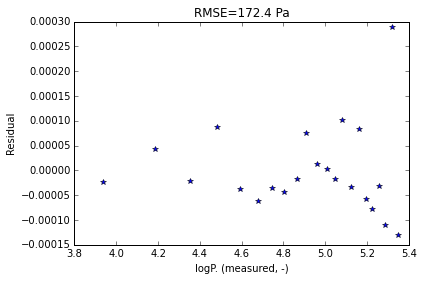These residuals look much better- no clear trends and much lower residual error. The Riedel equation is a better fit than the polynomial

## Nonlinear Regression¶

Regress P vs T data to the Antoine equation:

$$\log\left(P\right)=A+\frac{B}{T+C}$$

We use scipy's curve_fit with a custom function coding in the Antoine equation. Note: Doing curve fitting with log-transformed observations violates the assumtion of identically-distributed errors

Define Antoine function, regress and print out best fit values of $A$, $B$, and $C$:

In :
def NLRfun(X,A,B,C):
T=X
logP=A+B/(T+C)
yhat=logP
return yhat

X=data['Temperature'].values
y=np.log10(data['Pressure'].values)
Beta0=[10, -2000, 0]
Beta_names=['A: ','B: ','C: ']

beta,covar = curve_fit(NLRfun,X,y,p0=Beta0)
for b in zip(Beta_names, beta):
print(b+'{:.2f}'.format(b))

A: 9.02
B: -1207.37
C: -52.77


Make plot of residuals, and also calculate the RMSE of the predicted pressures:

In :
yhat=NLRfun(X,*beta)
plt.plot(y,y-yhat,'b*')
plt.xlabel('logP. (measured, -)')
plt.ylabel('Residual, log(Pa)')
plt.title('RMSE=%.1f Pa' %(np.sqrt(sum((10**y-10**yhat)**2))))

Out:
<matplotlib.text.Text at 0x7fe925f3cfd0>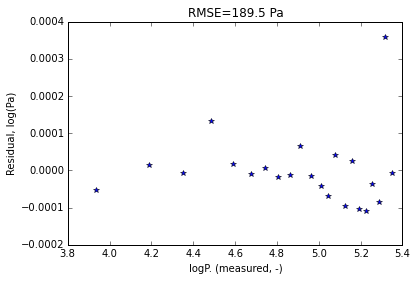Try the regression again without the log-transformed pressure observations

In :
def NLRfun(X,A,B,C):
T=X
P=np.exp(A+B/(T+C))
yhat=P
return yhat

X=data['Temperature'].values # Temperature and pressure are changed to column vectors
y=data['Pressure'].values
Beta0=[10, -2000, 0]
Beta_names=['A: ','B: ','C: ']

beta,covar = curve_fit(NLRfun,X,y,p0=Beta0)
for b in zip(Beta_names, beta):
print(b+'{:.2f}'.format(b))

A: 20.81
B: -2797.54
C: -51.85

In :
yhat=NLRfun(X,*beta)
plt.plot(y,y-yhat,'b*')
plt.xlabel('Press. (measured, Pa)')
plt.ylabel('Residual, Pa')
plt.title('RMSE=%.1f Pa' %(np.sqrt(sum((y-yhat)**2))))

Out:
<matplotlib.text.Text at 0x7fe925e61390>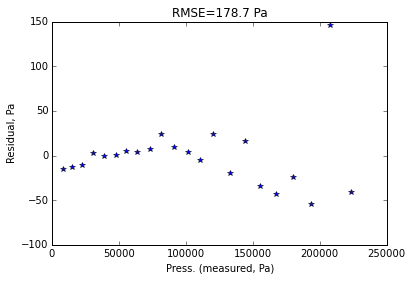Here, the residuals look similar to the log-transformed case, but the scale is different, and in terms of obervation error, the RMSE is lower without fitting the log-transformed pressure observations. The best fit values of $A$ and $B$ have also changed significantly.

# Conclusion¶

We have gone through many calculations common to chemical engineering (optimization, regression, integration) using Python. Hopefully this has been an educational excersise in presenting Python as an accessable tool to quickly analyze data and models. Feel free to download and edit this workbook as appropriate for your own needs!